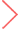24小时热闻

# 详解基于凸集投影方法的聚类算法

51CTO 2023-05-10 14:51:07### 凸集投影

#### 交替凸集投影#### 平行凸集投影### 基于凸集投影方法的聚类算法### 实验结果### 示例代码

``pip install pocs-based-clustering``

``#导入包 import time import matplotlib.pyplot as plt from sklearn.datasets import make_blobs from pocs_based_clustering.tools import clustering # 生成一个简单的数据集 num_clusters = 10 X, y = make_blobs(n_samples=5000, centers=num_clusters, /  cluster_std=0.5, random_state=0) plt.figure(figsize=(8,8)) plt.scatter(X[:, 0], X[:, 1], s=50) plt.show()````# 基于凸集投影方法的聚类算法 centroids, labels = clustering(X, num_clusters, 100) # 显示结果 plt.figure(figsize=(8,8)) plt.scatter(X[:, 0], X[:, 1], c=labels, s=50, cmap='viridis') plt.scatter(centroids[:, 0], centroids[:, 1], s=100, c='red') plt.show()``5.5亿用户的选择